﻿ Recycle-based Concrete: Modeling ResistanceInternational Journal of Composite Materials

p-ISSN: 2166-479X    e-ISSN: 2166-4919

2019;  9(2): 39-43

doi:10.5923/j.cmaterials.20190902.02### Recycle-based Concrete: Modeling Resistance

Serifou Mamery Adama, Jolissaint Obre Sery Paul, Ouattara Souleymane, Emeruwa Edjikémé

Department of Earth Sciences and Mineral Resource, Geomaterial Laboratory, University of Félix Houphouët-Boigny, Côte d’Ivoire

Correspondence to: Serifou Mamery Adama, Department of Earth Sciences and Mineral Resource, Geomaterial Laboratory, University of Félix Houphouët-Boigny, Côte d’Ivoire.
 Email: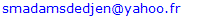Abstract

This study aims to valorize non-biodegradable waste through their recycling as aggregates in concrete. Concretes made from different types of recyclates (recycled concrete, glass and tire) and natural aggregates were made and tested in compression at 28 days. A modeling test of the resistance of the various concretes to compression has been carried out. Concretes of natural granulate, recycled concrete and crushed glass have a resistance that decreases slightly with the percentage of aggregates. It is also noted that the concrete of natural granulate and recycled concrete have substantially equal strengths. Contrariwise, the glass concrete gives a lower average strength and the used tire concrete is well below the other recyclates. The compressive strength of concrete follows an exponential mathematical law that takes into account the volume fraction, the density, and the particle size. Thus, the application and the compressive strength show that concretes based on recycled concrete can be used in construction in place of those made from natural aggregates.

Keywords: Concrete, Recyclates, Resistance, Glass, Tire, Recycled concrete

Cite this paper: Serifou Mamery Adama, Jolissaint Obre Sery Paul, Ouattara Souleymane, Emeruwa Edjikémé, Recycle-based Concrete: Modeling Resistance, International Journal of Composite Materials, Vol. 9 No. 2, 2019, pp. 39-43. doi: 10.5923/j.cmaterials.20190902.02.

### 1. Introduction

The problematic of waste management is a stake issue for the state of Côte d'Ivoire. This is why the promotion of waste recovery remains a challenge for the authorities. Waste from organic materials is used as compost in agriculture, the problem remains with non-biodegradable waste such as used tires, recycled concrete and bottled glasses. One possibility is to use this waste as aggregates in concrete. Indeed, some authors such as [1-5] used glasses as a substitute for natural aggregates. Other authors have also used tire grinding . This study is therefore part of this approach. It aims to use three types of non-biodegradable waste (crushed glass, recycled concrete and tires) in the concrete formulation. To do this, a modeling of the compressive strength of the various concretes has been carried out.

### 2. Materials and Methods

#### 2.1. Materials

This study focuses on three types of waste: glass bottles, recycled concrete and used tires recovered in the district of Abidjan. The bottles and cement were crushed with a mass and the tires were cut with a saw.

#### 2.2. Methods

Ÿ Concrete Formulation
This waste was incorporated into concrete to replace 100% gravel.
For the same matrix, the influence of the volume of agregates was studied for all the mixing compositions. Thus, only the percentage of recyclates increased from 20 to 50%. The table shows the different proportions used.
 Table 1. Composition of mixtures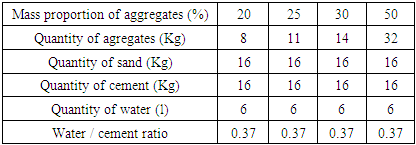For the realization of our test, we weigh the components including the water in the following order: gravel, sand, cement. The raw materials are mixed dry, then the mixing water is poured in order to obtain the fresh concrete.
The specimens (16 x 32) are filled in accordance with the P 13-451 standard, in two identical layers, each being vibrated with a 25 mm vibrating needle. The test pieces are demolded the next day (24 hours) from their manufacture, they are then marked and dated. Conservation is done in a wet pond until maturation (28 days). The compressive strength of the concrete obtained is then measured on three samples of each composition. Similarly, resistance measurements of the various concretes were made with a CONROLAB IGM hydraulic press with a maximum capacity of 1500 kN and a loading speed of 5 kN / s. The compressive strength was determined on cylindrical concrete specimens, following the French standard NF P18-406.
A comparison of the resistance values obtained from the different concretes made it possible to establish a law giving the resistance as a function of the characteristics and the volume fraction of aggregates.

### 3. Results and Discussion

#### 3.1. Mechanical Resistance

Figure 1 and table 2 illustrate the strength results obtained on the various concretes.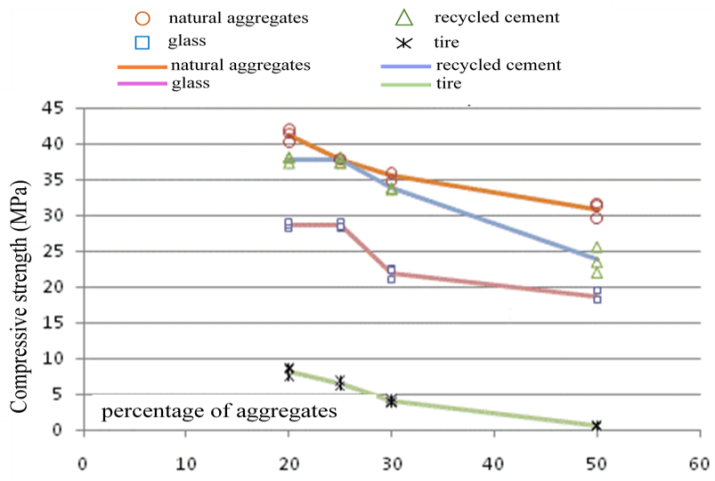Figure 1. Resistance of the different mixtures according to the masspercentage of aggregates
 Table 2. Resistance to the compression test of different concretes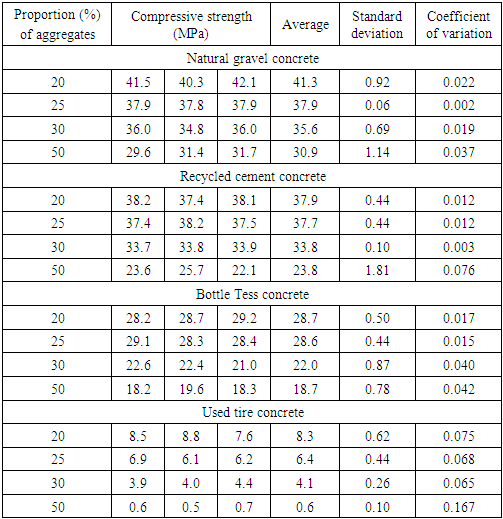The graph of figure 1 shows the resistances obtained as a function of the mass percentages of the different types of concretes. There is clearly a decrease in strength according to the percentage of aggregates regardless of the material and the type of granulate. This decrease can be linked to the low adhesion at the interface aggregates (aggregates) -mortier (cement matrix). Indeed, recycled aggregates such as glass and tire have smooth and non-absorbent surfaces, which does not promote good fixing of the mortar. The application of a stress will cause a rapid detachment of the mortar, hence the decline in resistance.
This decline in strength associated with the incorporation of recycled aggregates into concrete was also found by  and .
However,  explain this decline in strength by the shape of glass aggregates.
Concretes based on recycled tires give the weakest resistances followed by glass concretes. It is noted that the decrease in resistance as a function of the increase in the rate of glass granulate is important. The strength of glass concrete is lower than that of natural gravel concrete. The incomplete adhesion of the glass to the cement paste is also the cause of this decrease in resistance. This result is consistent with those obtained by [10,11]. In addition, this decrease in strength due to the increase in the proportion of aggregates could also be explained by the increase in the volume occupied by aggregates. As the volume occupied by the aggregates increases, the amount of mortar becomes insufficient to bind the aggregates properly. This induces the creation of granulate-aggregate contact zones. The area of contact of aggregates is a zone of weakness through which the crack spreads.
However, the strength at low percentage is higher because the amount of mortar is important to form a good matrix around the aggregates.
In addition, values close to the strengths of recycled concrete and natural granulated concretes are explained by a better adhesion of the cured cements to the matrix.
Table 2 shows a low dispersion of the resistances around the mean value (standard deviation between 0.10 and 1.81). The values obtained are significant and can be used to determine the influence of the type of aggregate and its size on the quality of the concrete.
Table 3 a and b presents the statistical analysis of the compression tests for each percentage of aggregates (average, type of deviation and coefficient of variation) (cov = type of deviation/average).
 Table 3. a) Mean compressive strengths, standard deviations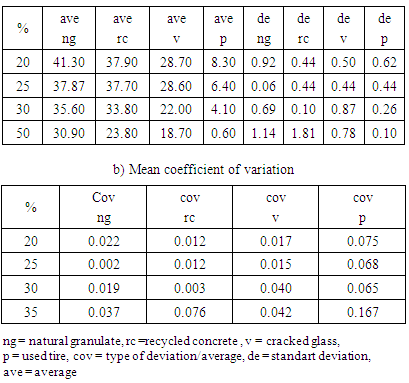This table shows that there is no relationship between the percentage of substitution and the standard deviations, whatever the type of aggregate. The dispersion of the values of the resistances is therefore not related to the proportion of granules. This confirms that the compressive strengths depend essentially on the proportion and type of aggregate in the concrete. Also, to determine the factor related to the proportion and type of granulate that most influences the strength of recycled concrete the following analyzes were performed.

#### 3.2. Influence of the Volume Ratio

The volume ratio is the percentage of granules per unit volume of the concrete. The higher the proportion of granulate, the higher the volume ratio. Therefore, the aggregates will tend to be in contact with each other. This explains the drop in resistance with the increase in the percentage of aggregate (see figure 1). To generalize this finding to different types of aggregates, their compressive strengths have been normalized (ie the resistance has been divided by the mean value). This normalization makes it possible to minimize the effect of the value of the resistance on the distribution of the curves. Figure 2 shows the normalized resistance curves as a function of percentage and type of aggregate.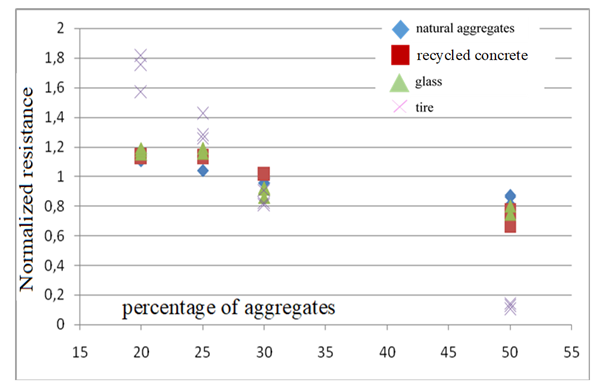Figure 2. Normalized resistance according to the percentage and type of aggregates
This figure indicates that the behavior of natural aggregates, recycled concrete and glass is almost identical without the tire granulate. This behavior can be characterized by a slope of the regression line. Table 4 gives the value of these slopes. It is noted that the recycled concrete and the glass have almost identical slopes (-0.014). Their volume ratios thus influence the compressive strength in a similar manner in the cement matrix. Contrariwise, with the natural granulate the line is practically horizontal (slope = -0.009) so the volume ratio has little influence on the compressive strength. However, the tire granulate has a very steep slope (-0,051) compared to the first three, its volume ratio influences more compressive strength.
 Table 4. Slopes of the standardized resistance according to the per centage of aggregates (% -1)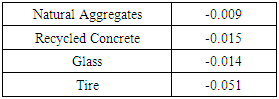#### 3.3. Proposition of a Law Giving Resistance

A line could fit on each series of points in Figure 3 giving for each material the resistance as a function of the percentage of aggregates. Thus, at 0% of aggregates, 4 values very different from the strength of the mortar would be obtained. Which is not logical because it is the same mortar. Also, to find a common resistance value of the mortar that adjusts with these different types of concrete, an exponential type model such as that proposed by  for tire aggregates is chosen. The model giving the resistance will therefore be of the form: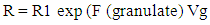(1)
In which F is a function that depends on the characteristics of the aggregates only (therefore of the type of aggregates), and Vg is the volume fraction of aggregates. The volume fraction was preferred to the mass fraction to design the mixtures because the density of the aggregates is different and the space occupied by each varies according to the density of each material. The advantage of this relationship is that it makes it possible to take into account the difference between the aggregates, the influence of the relative quantity of aggregates and to have for Vg = 0 a common value. Figure 3 shows the curves obtained.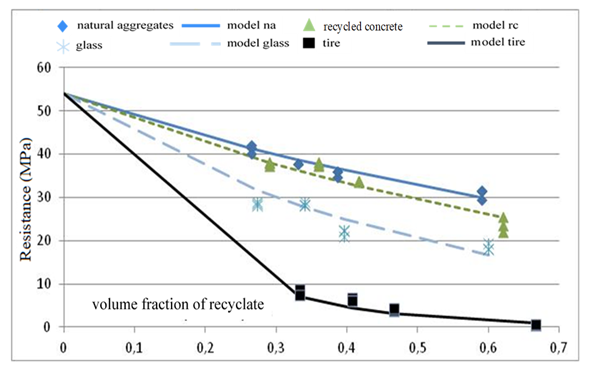Figure 3. Model of variation of the resistance as a function of the volume fraction
Figure 3 gives the value of the strength of the mortar: R1 = 54 MPa. It is the value that minimizes the gap between the model and the experimental points. The function F therefore depends only on the characteristics of the aggregates. The value of F is obtained by making a filter with an exponential function. Table 5 presents the values of F and the values of the characteristics of each material (Young's modulus, density and Weibul parameters). The structure of the data in Table 4 is particular, indeed the set of 4 values is divided into three values (natural gravel, recycled concrete, glass) and the tire further away. As a result, all values are correlated with each other.
 Table 5. Characteristic values of aggregates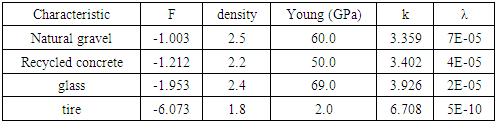According to , the relative values of the Young's modulus of aggregates with respect to that of the sand are involved in the resistance. Indeed, when we compress the material, we do not impose the same deformation to the aggregate and mortar or sand. If the module gap is large therefore microcracks form around the aggregates, which forms as much crack initiation. On the other hand, if the difference in density between the granulate and the sand is too much, segregation and formation of aggregates will occur. Clusters are places of stress concentration, so privileged places to initiate cracks. Thus, the function F depends on the density and Young's moduls. Moreover, the compactness also intervenes in the resistance. However, the compactness is related to the particle size curves and also to the coefficients which characterize it (percentiles and coefficients k and lambda of the law of Weibul). Figure 4 presents an example of comparison between the Weibul model and the experimental data for natural gravel.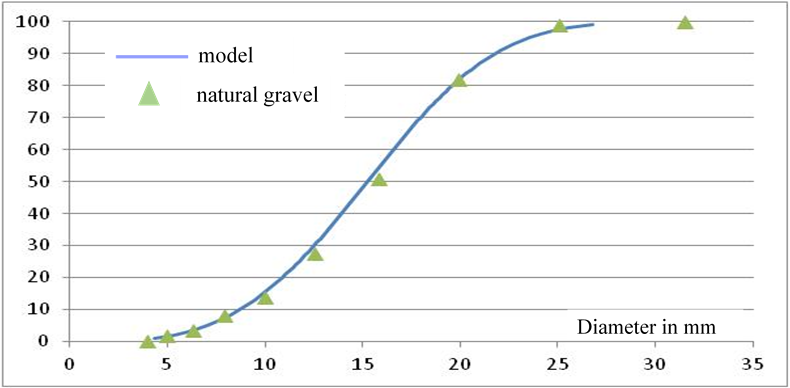Figure 4. Comparison between the Weibull model and the experimental data for granulometry of natural gravel
Overall, adjustment Weibul's law fit on granulometry curves is very good for aggregates (R2 = 0.99) but a little less good for sand (R2 = 0.93). Consequently, the values of k, ln (λ) make it possible to express the function F. Figures 5, 6 and 7 show the variation of F as a function of ln (λ), k and (1-dg / ds) where ds is the density of the sand and dg the density of the recycled gravel.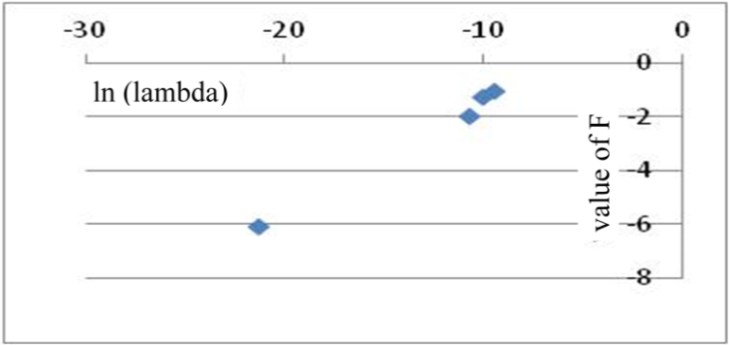Figure 5. Variation of F with the logarithm of the parameter of scale λ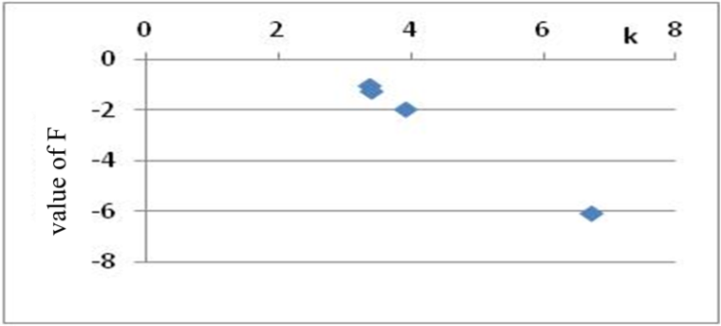Figure 6. Variation of F with the logarithm of the form factor k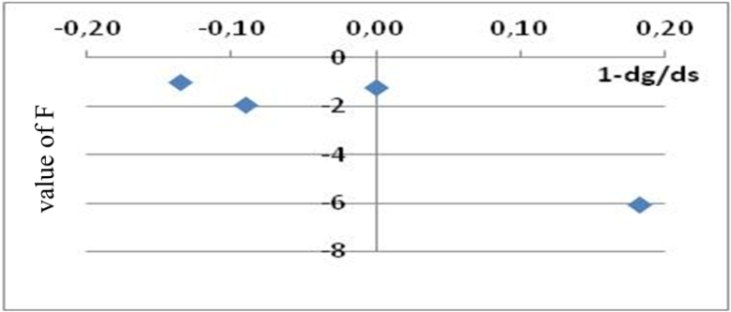Figure 7. Variation of F with the relative density (1-dg / ds) of the recycled aggregate with respect to the sand
All these figures show that F can be written in the form of an affine function of these different factors. Thus, none of these factors seems to dominate the value of resistance. A general relation of F can thus be written of the form: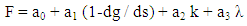(2)
Ÿ Discussion
We searched for a universal model explaining the variations in compressive strength as a function of the material and the volume fraction of aggregates. The fact that the model fits well on the experimental data is of course no proof that it is good and universal. The dependence in the form of an exponential function with Vg crosses results from the bibliography , and makes it possible to separate what appears from the type of granulate and what emerges from the volume fraction. Figure 8 shows the comparison between the model and the experimental results. The value of 54 MPa for the mortar (Vg = 0) seems high. On the other hand, the experimental data do not allow to distinguish what relates to the granulometry and what is the material. Other tests with the same materials and different particle sizes would validate or invalidate the tracks proposed in this study. The bibliography also indicates a dependence with the Young's modulus if that of the granulate is very different from that of the sand. This could not be highlighted in this study.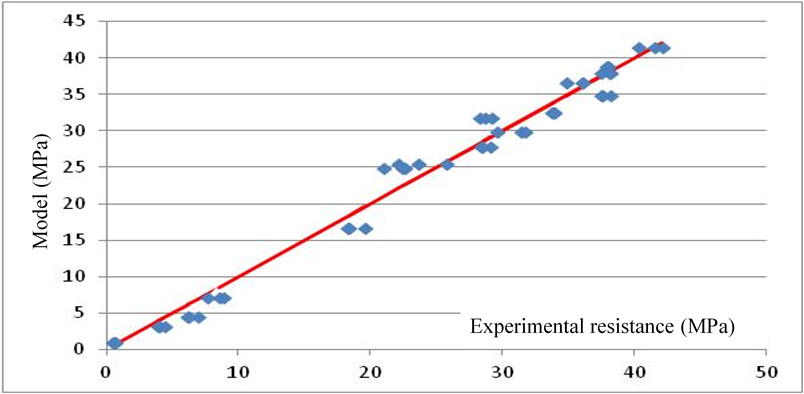Figure 8. Comparison of the experimental resistance and the resistance given by the model for the 4 materials

### 4. Conclusions

Tests were carried out on concretes of different compositions containing aggregates from recycling at 28 days. These recycled aggregates are crushed glass, recycled crushed concrete and used tire. Compression strength tests were carried out with concretes containing different percentages of aggregates. The percentage of aggregate used has more influence on used tire concrete where there is a sharp decrease in compressive strength. Concrete aggregates, recycled concrete and crushed glass have a resistance that decreases slightly with the percentage of aggregates. If we look at the type of aggregate, we see that the natural aggregate and the recycled concrete have substantially equal strengths. Glass gives lower average strength and used tire concrete is well below other recyclates. In addition, the compressive strength was modeled using an exponential law that allows to take into account the volume fraction of material, density and particle size, which allows to predict the behavior of concrete based of recyclates.
However, the ecological impact that has not been studied (carbon footprint for example) would show the interest of recycling this waste. The use of waste in concrete will provide benefits in the disposal of waste and, in addition, preserve the environment from damage due to the use of natural resources as aggregates.

### References

  Rachida I., Martin C., Arezki T (2010). Etude des propriétés des bétons de verre. Technologie valorisation Vol. 16 N°5/6, pp 70-77.  Jin C., Meyer C. et Baxter S. (2000). “Glasscrete”-Concrete with glass aggregate, American concrete institute, Material Journal, n°97, pp.208-213.  Newlands, Dyer, T.D. (2005). Achieving Sustainability in Construction, Proceedings of the International Conference Held at the University of Dundee, Scotland, UK on 5-7 July.  Meyer, C., Baxter, S. et Jin, W (1996). Alkali-silica reaction in concrete with waste glass as aggregate, Materials for a New Millennium, Proceedings of ASCE Materials Engineering Conference, Washington, pp. 1388–1394.  Lee G., Ling T.C., Wong Y.L., Poon C.S. (2011). Effects of crushed glass cullet sizes, casting methods and pouzzolanic materials on ASR of concrete blocks. Construction and Building Materials, 25, pp 2611-2618.  Siddique, Rafat and Naik, Tarun R. (2004). Properties of concrete containing scrap-tire rubber - an overview - Waste Management - Vol. 24 - Numéro 6, pp. 563-569.  Tung-Chai L., Chi-Sun P., et Shi-Cong K. (2011). Influence of recycled glass content and curing conditions on the properties of self-compacting concrete after exposure to elevated temperatures. Cement & Concrete Composites, 18 p.  Kou S-C. et Poon C-S. (2009). Properties of self-compacting concrete prepared with recycled glass aggregate. CemConcr Compos 31(2), pp 103–107.  Polley C., Cramer S.M. et Rodolfo V (1998). Potential for using waste glass in Portland cement concrete, Journal of Materials in Civil Engineering, vol. 10, n° 4, pp 210-219.  Topçu I. B. et Mehmet C (2004). Properties of concrete containing waste glass. Cement and Concrete Research 34, pp 267–274.  Topçu I., Boga A., Bilir T. (2008). Alkali–silica reactions of mortars produced by using waste glass as fine aggregate and admixtures such as fly ash and Li2CO3. Waste Manage 2008;28(5):878–84.  Benazzouk, A., Douzane, O., Langlet, T., Mezreb, K., Roucoult, J. M., and Quéneudec, M. (2007). Physico-mechanical properties and water absorption of cement composite containing shredded rubber wastes - Cement and Concrete Composites - Vol. 29 - Numéro 10-pp. 732-740.  Ganijian, Eshmaiel, Khorami, Morteza, and Maghsoudi, Ali Akbar (2009). Scrap-tyre-rubber replacement for aggregate and filler in concrete - Construction and Building Materials - Vol. 23 - Numéro 5- pp. 1828-1836.